# How to Calculate and Solve for Modulus of Elasticity of Composites Lower Bound | Composites

The modulus of elasticity of composites lower bound is illustrated by the image below.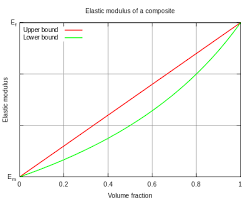To compute for modulus of elasticity of composites lower bound, four essential parameters are needed and these parameters are Modulus of Elasticity of the Matrix (Em), Modulus of Elasticity of the Particle (Ep), Volume Fraction of the Matrix (Vm) and Volume Fraction of the Particle (Vp).

The formula for calculating modulus of elasticity of composites lower bound:

Ec(l) = EmEp/VmEp + VpEm

Where:

Ec(u) = Modulus of Elasticity of Composites Lower Bound
Em =Modulus of Elasticity of the Matrix
Ep = Modulus of Elasticity of the Particle
Vm = Volume Fractions of the Matrix
Vp = Volume Fractions of the Particle

Let’s solve an example;
Find the modulus of elasticity of composites lower bound when the modulus of elasticity of the matrix is 2, the modulus of elasticity of the particle is 6, the volume fractions of the matrix is 8 and the volume fractions of the particle is 4.

This implies that;

Em =Modulus of Elasticity of the Matrix = 2
Ep = Modulus of Elasticity of the Particle = 6
Vm = Volume Fractions of the Matrix = 8
Vp = Volume Fractions of the Particle = 4

Ec(l) = EmEp/VmEp + VpEm
Ec(l) = (2)(6)/(8)(6) + (4)(2)
Ec(l) = (12)/(48) + (8)
Ec(l) = (12)/(56)
Ec(l) = 0.214

Therefore, the modulus of elasticity of composites lower bound is 0.214 Pa.

Nickzom Calculator – The Calculator Encyclopedia is capable of calculating the modulus of elasticity of composites lower bound.

To get the answer and workings of the modulus of elasticity of composites lower bound using the Nickzom Calculator – The Calculator Encyclopedia. First, you need to obtain the app.

You can get this app via any of these means:

You can also try the demo version via https://www.nickzom.org/calculator

Apple (Paid) – https://itunes.apple.com/us/app/nickzom-calculator/id1331162702?mt=8
Once, you have obtained the calculator encyclopedia app, proceed to the Calculator Map, then click on Materials and Metallurgical under Engineering.Now, Click on Composites under Materials and Metallurgical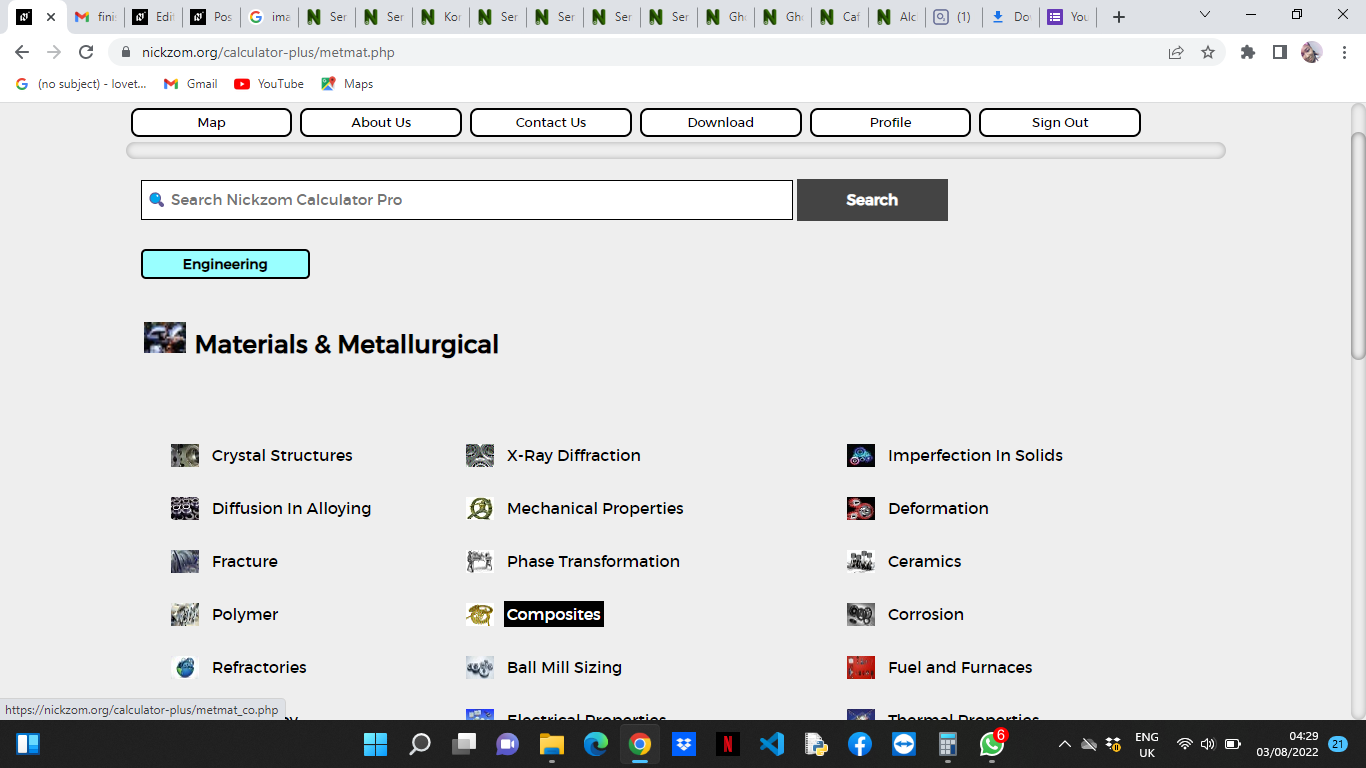Now, Click on Modulus of Elasticity of Composites Lower Bound under Composites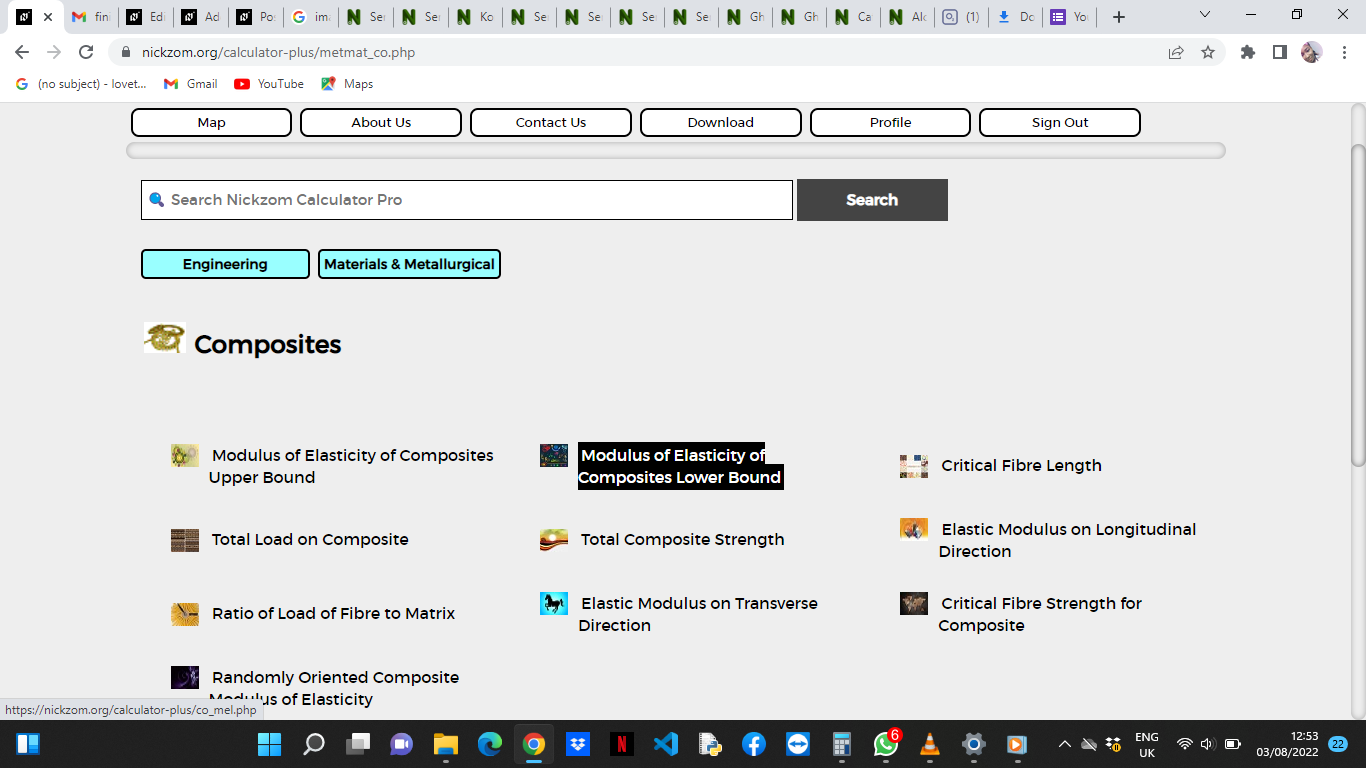The screenshot below displays the page or activity to enter your values, to get the answer for the modulus of elasticity of composites lower bound according to the respective parameter which is the Modulus of Elasticity of the Matrix (Em), Modulus of Elasticity of the Particle (Ep), Volume Fraction of the Matrix (Vm) and Volume Fraction of the Particle (Vp).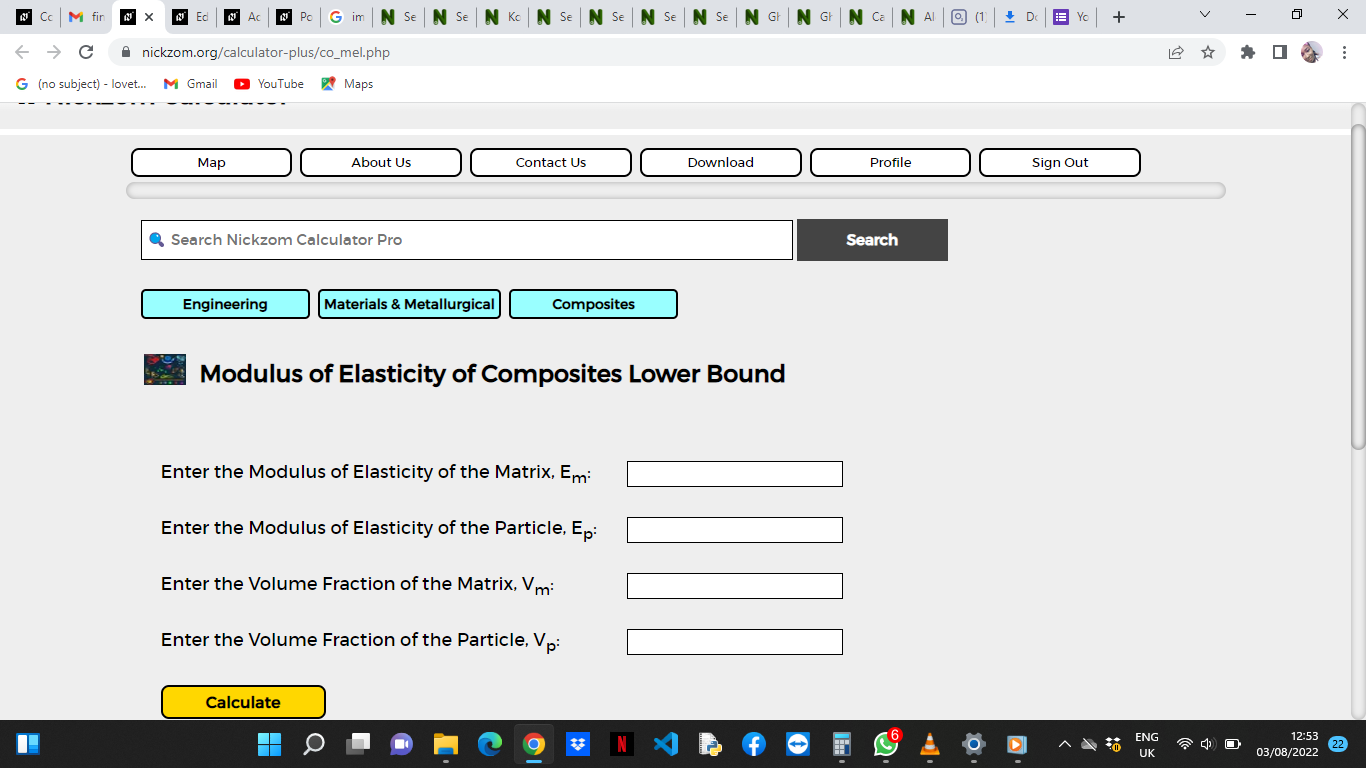Now, enter the values appropriately and accordingly for the parameters as required by the Modulus of Elasticity of the Matrix (Em) is 2, Modulus of Elasticity of the Particle (Ep) is 6, Volume Fraction of the Matrix (Vmis 8 and Volume Fraction of the Particle (Vp) is 4.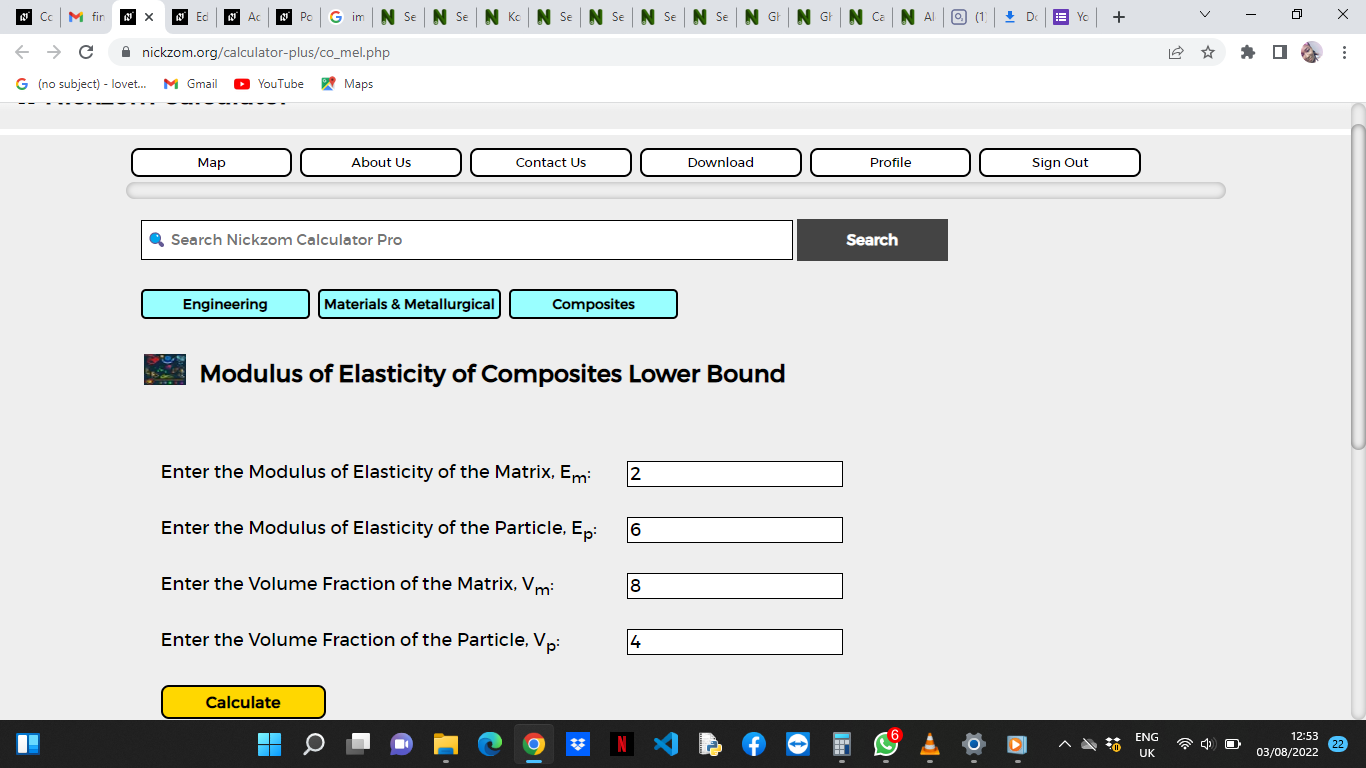Finally, Click on Calculate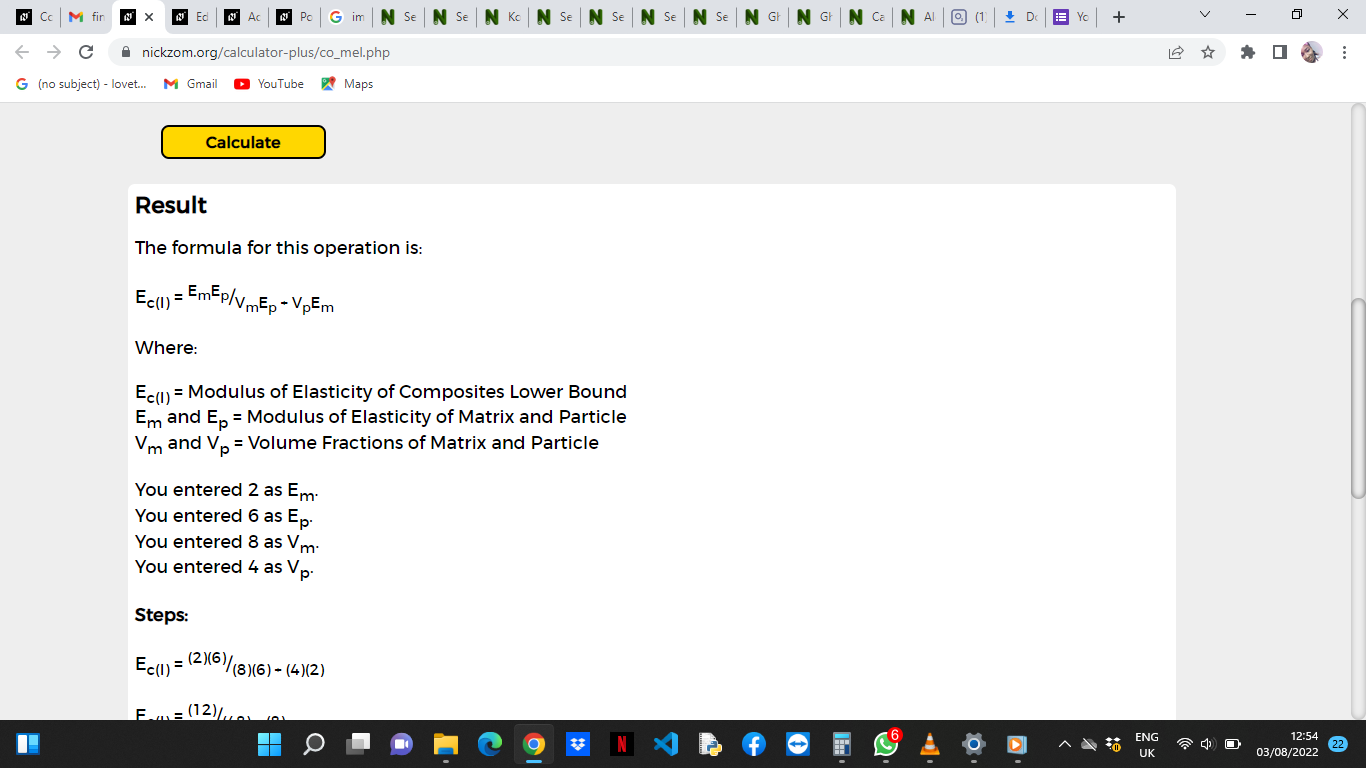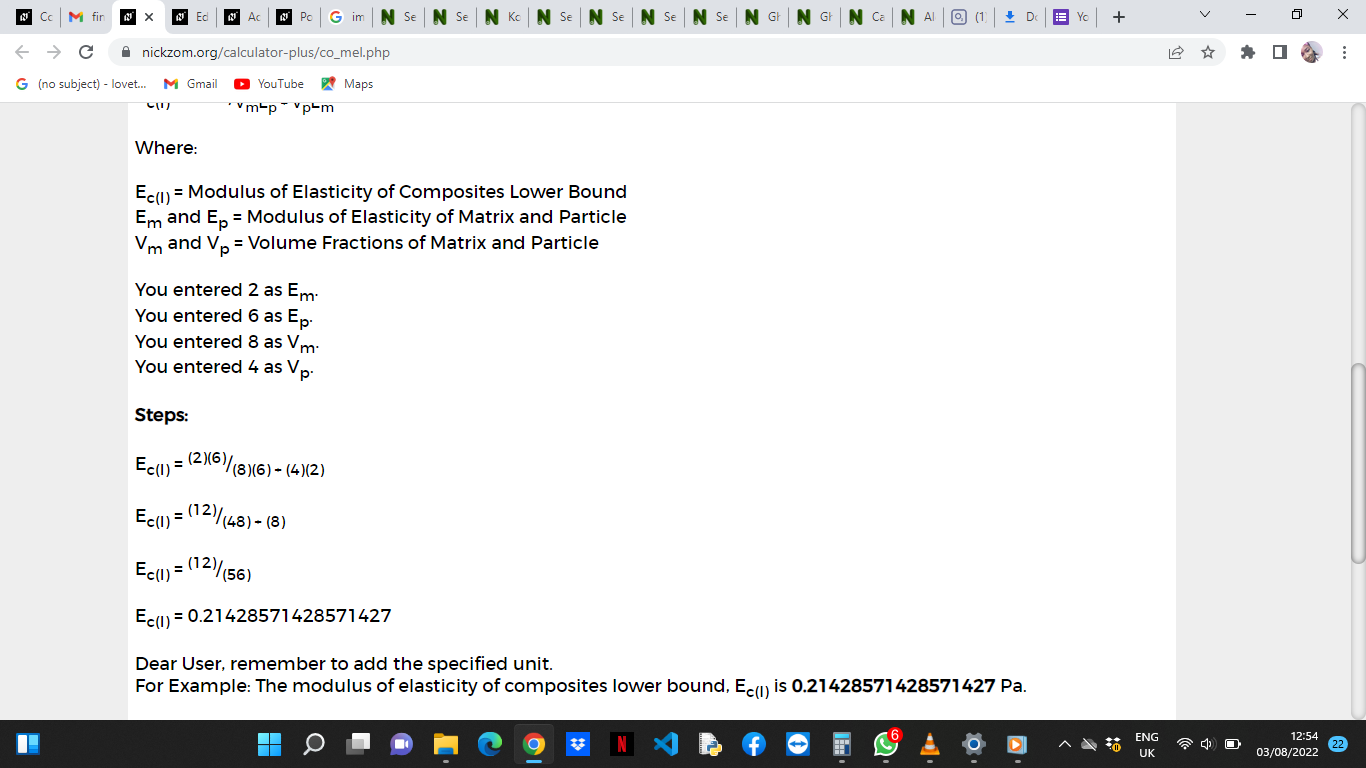As you can see from the screenshot above, Nickzom Calculator– The Calculator Encyclopedia solves for the modulus of elasticity of composites lower bound and presents the formula, workings and steps too.